# Team:HUST-China/Gene Pathway

Gene Pathway | iGEM HUST-China

# PART1 Pre-Analysis

The construction of the model is based on the analysis of the scale of reaction speed, Michaelis-Menten equation and major chemical equation, which is shown in the figure.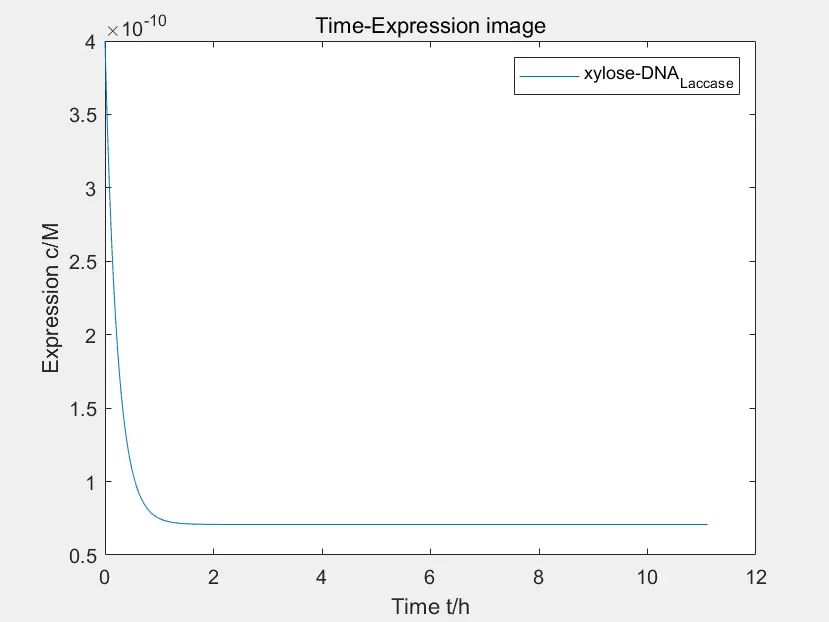Figure 1: the time-express image of xylose activating P_YNR071C

The data in the figure is calculated when the concentration of Pichia pastoris is $$4*10^{-10}M$$

This is the time-express image of $$xylose$$ activating $$P_{YNR071C}$$. We first assume that all promoters are activated, and then we can see through the image. After about 1H, the activation reaction reaches equilibrium,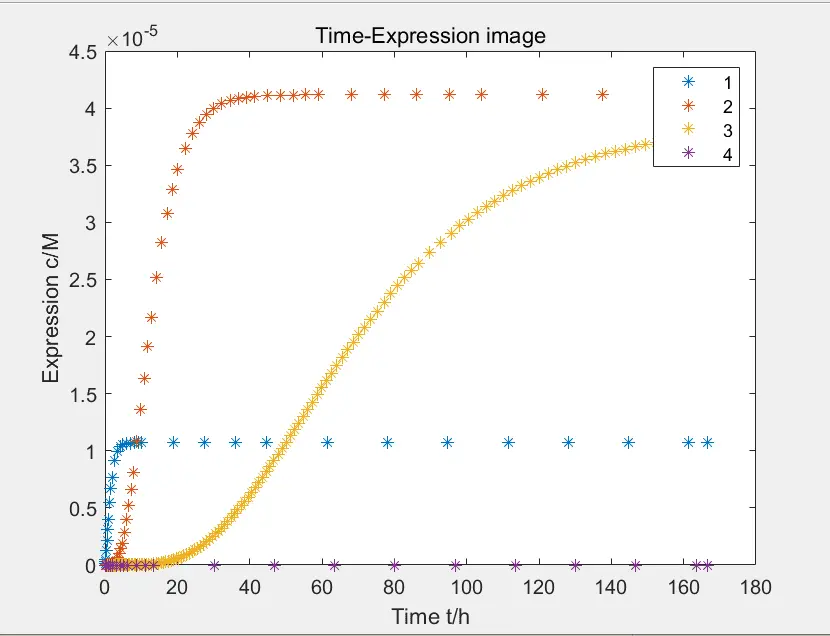Figure 2: the yield of each pathway

The data in the figure is calculated when the concentration of Pichia pastoris is $$4 * 10 ^ {- 10} M$$

Above is the yield of each pathway. The yield of each pathway is one or two order of magnitude higher than that when gene is activated Therefore, reactions of gene activation can be simulated by separating fast and slow scales, that is, the gene activation is assumed to be in a balanced state during product expression: $$\frac{d[xylose-DNA]}{dt}=0\\ \frac{d[DNA]}{dt}=0$$

Then we get $$[xylose-DNA]=\frac{K[DNA_{all}]}{K+\frac1{[xylose]}}$$

The inhibition of $$P_{anb1}$$ can also be simplified by this formula, which is $$[DNA]=\frac{[DNA_{all}]}{K{[R]}+1}$$. At the same time, we also simplified the Michaelis-Menten equation for the enzyme reaction in each pathway, and simulated each pathway by ordinary differential equation.

# PART2 Evolution Process of the Pathways

Our team established the chemical rate equation system through deduction of the major chemical equation, and simulated the statistical average evolution process of the pathways as follows: $$\frac{dx(1)}{dt}=k_1DNA-\delta_1x(1)$$, $$\frac{dx(2)}{dt}=k_2x(1)-\delta_2x(2)$$

This is a simple ordinary differential equation simulation of protein expression. Since the reactions are first-order reactions, it can be seen from the major chemical equation that x is the expected yield of product, where $$X_1$$is the expression of mRNA and $$X_2$$is the expression of protein.

The analytical solution can be calculated: $$p=\frac{DNA*k_1k_2}{\delta_1-\delta_2}[\frac{\delta_1-\delta_2}{\delta_1\delta_2}-(\frac{e^{-\delta_1t}}{\delta_1}+\frac{e^{-\delta_2t}}{\delta_2})]$$

Its derivation is $$\dot p=\frac{DNA*k_1k_2}{\delta_1-\delta_2}(e^{-\delta_1t}+e^{-\delta_2t})$$

The yield at equilibrium and maximum yield are $$\frac{DNAk_1k_2}{\delta_1\delta_2}$$, and $$\delta_1$$ and $$\delta_2$$ are inversely proportional to it. Assume $$\delta_1$$ and $$\delta_2$$ is in the same order of magnitude, and $$\delta_1$$ is always greater than $$\delta_2$$. It can be seen that the $$\delta_1$$ is inversely proportional to the expression rate of $$p$$, which has the opposite effect of the DNA concentration and $$k_1, k_2$$. Our design for pathway took this as a reference.

If $$\varepsilon(\frac1{\delta_2}-\frac1{\delta_1})=e^{-\delta_1t}+e^{-\delta_2t}$$, we can get implicit solution:

It can be seen from the above formula that whether the time of the maximum expression rate or the time reaching equilibrium, only $$\delta_1 and \delta_2$$ matter. We solved the following problems, as shown in the figure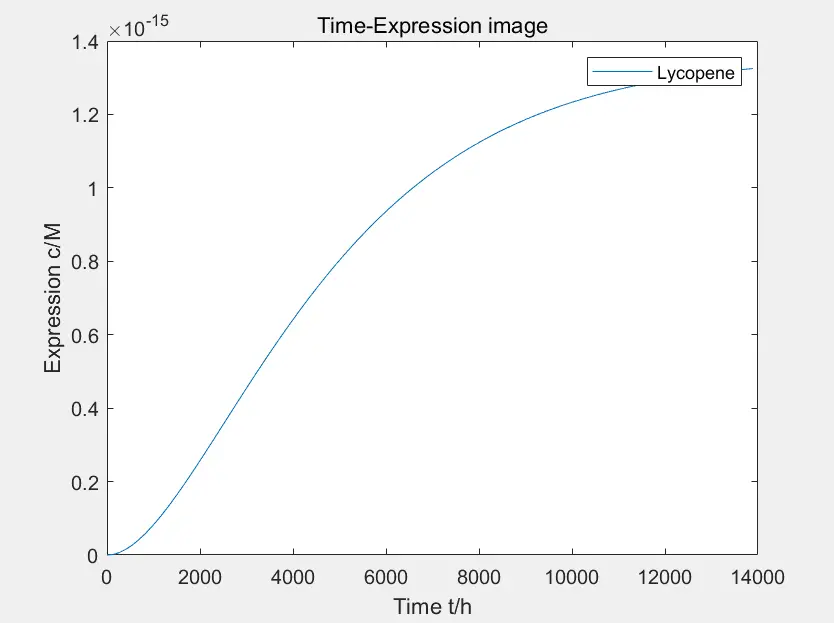Figure 3: the prototype of lycopene expression

The data in the figure is calculated when the concentration of Pichia pastoris is $$4 * 10 ^ {- 10} M$$

This is the prototype of lycopene expression constructed by the team. It can be seen that the product expression of this pathway is lower and more time-consuming compared with other pathways. There are many intermediate reaction steps to synthesize lycopene in lycopene pathway, thus the degradation of intermediate products will slow down the final synthesis speed and affect the yield, as shown in the figure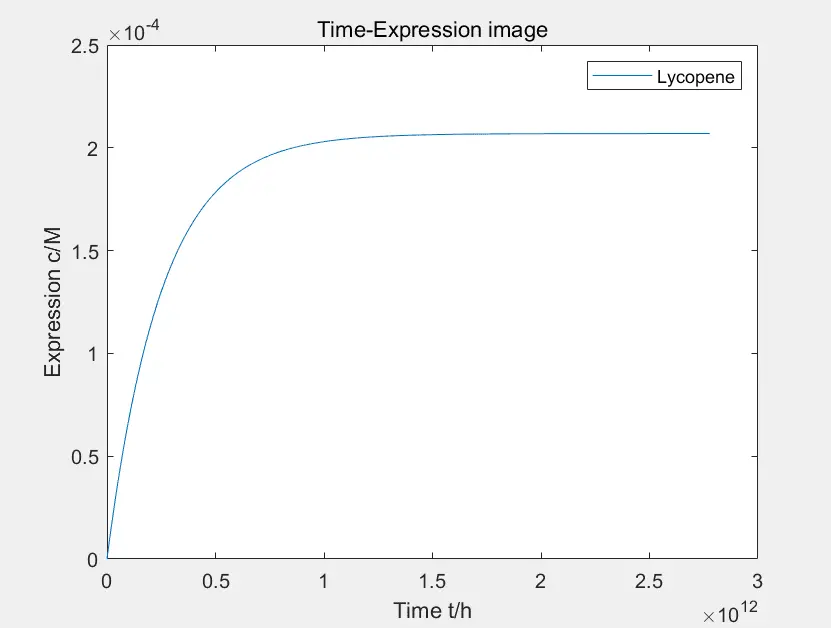Figure 4: the prototype of lycopene expression

The data in the figure is calculated when the concentration of Pichia pastoris is $$4 * 10 ^ {- 10} M$$

By reducing the degradation rate of intermediate products, the yield will increase at the cost of prolonging the time, but the reaction rate is not accelerated here. Through the comparison after adjusting parameters, we found that changing the degradation rate has no effect on the yield of the ongoing reaction at the same time, only influencing the yield at equilibrium. In conclusion, in the lycopene expression system, the final yield can only be controlled by controlling the degradation of intermediates, and this method won't affect the reaction rate.

In order to solve the problem of slow reaction, we discussed the problem of reaction steps. First, we know that the steps of reaction will affect the speed, as shown in the figure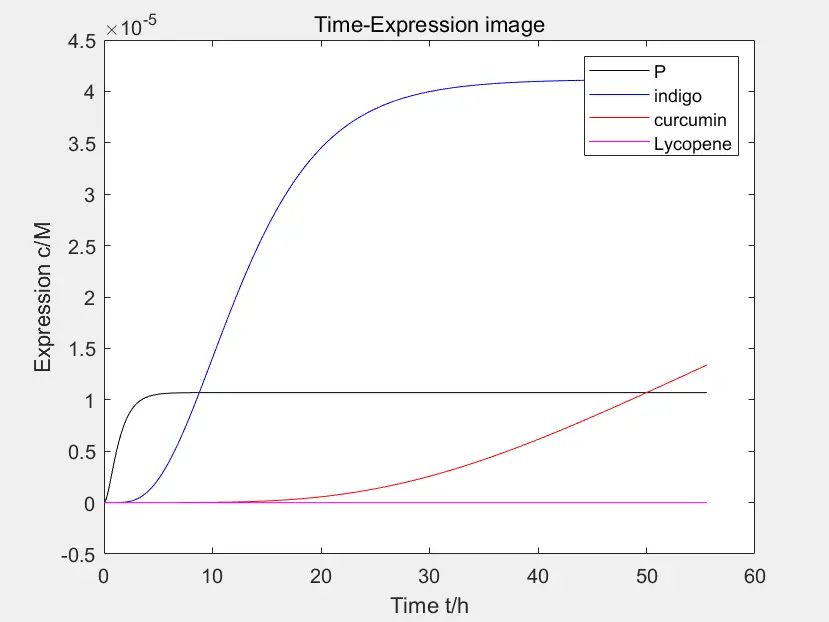Figure 5: parallel comparison of the four pathways

The data in the figure is calculated when the concentration of Pichia pastoris is $$4 * 10 ^ {- 10} M$$

This is a parallel comparison of the four pathways in the early stage of the project. Among them, the first pathway expressing the peptides has high rate of expression and quickly reaches equilibrium. The expression rate of pathway 2 is slower than that of pathway 3. The different speed contradicts with the desired final effect of "producing sufficient hair dye paste by fermentation within a certain time, and obtaining different colors by mixing". After consulting essays, it is known that curcumin can be synthesized by the reactions shown in the figure.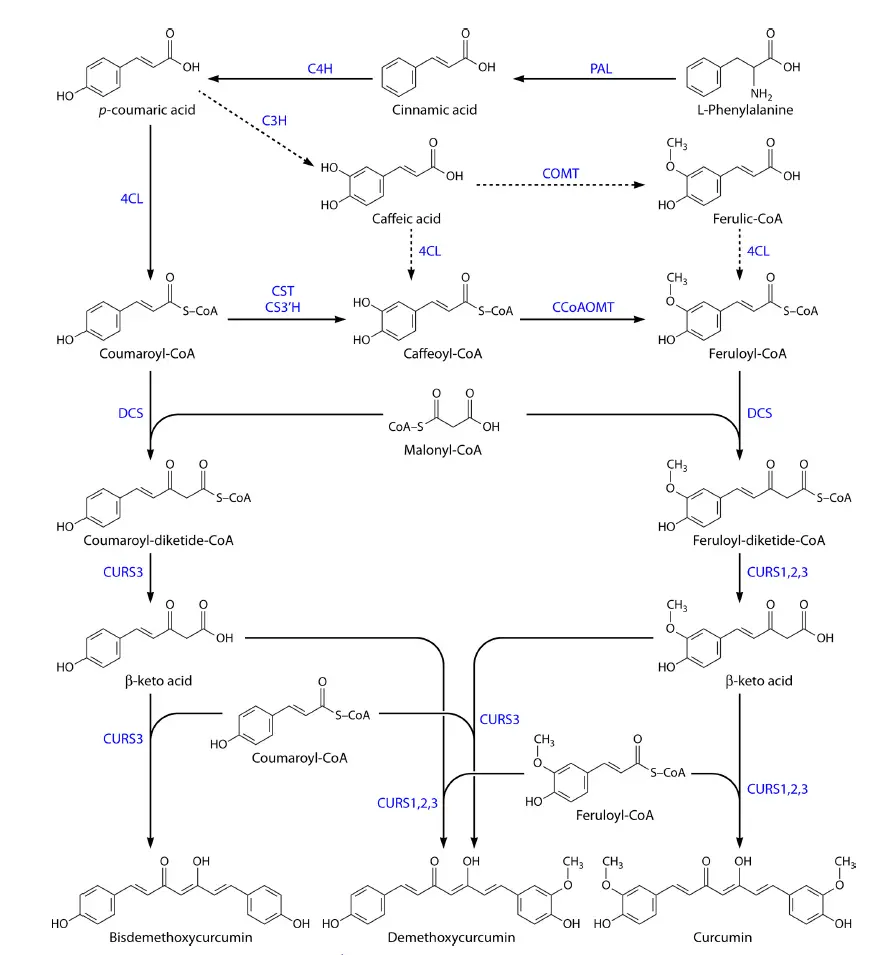Figure 6: the reactions

Redesigned of the project after comparing those parameters.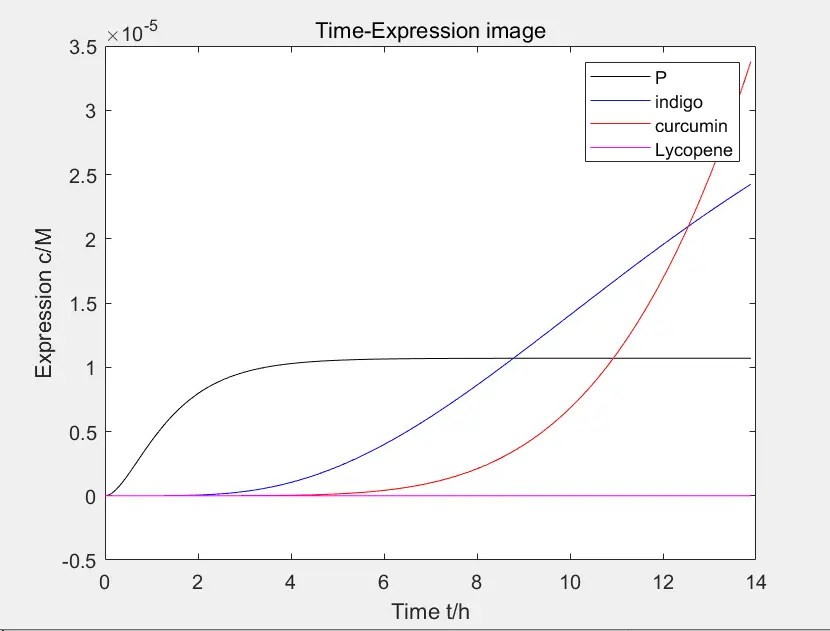Figure 7: the movement of the intersection

The change of reaction can be observed by the movement of the intersection of path 1 and path 3, as shown in the figure

# PART3 Activator and Inhibitor

As for the problem of binding of activator and inhibitor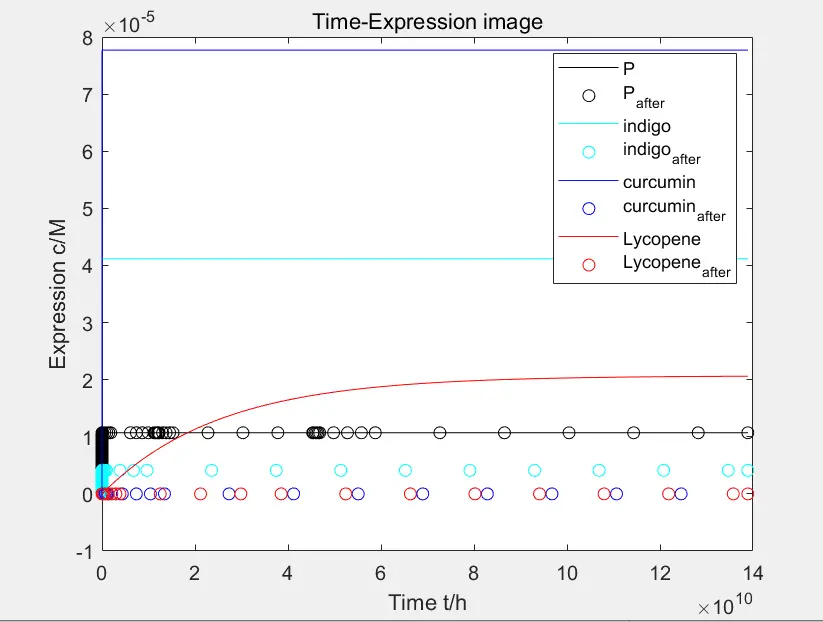Figure 8: expression after adding xylose to the third and fourth pathways

In the reaction pathway, after adding xylose to the third and fourth pathways, the expression of the product is significantly inhibited. The inhibition effect is not particularly ideal in the second pathway. In the first pathway, the peptide expression is almost unaffected because there is no substance designed to eliminate peptides.

Next is the binding of xylose and promoter.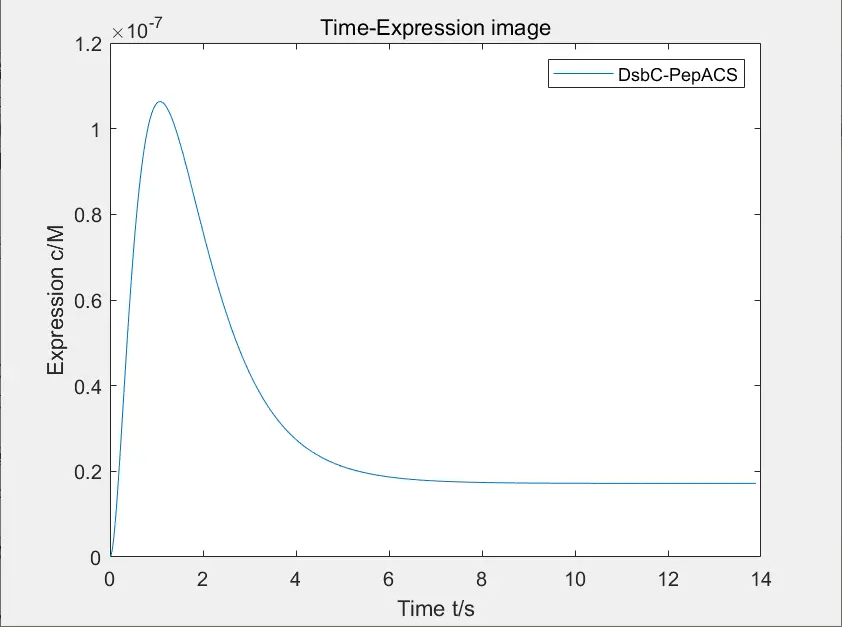Figure 9: expression of disulfide isomerase and polypeptide complex

The figure below shows the image of expression of disulfide isomerase and polypeptide complex after adding xylose, and the figure below shows the change of concentration of DNA bound to xylose.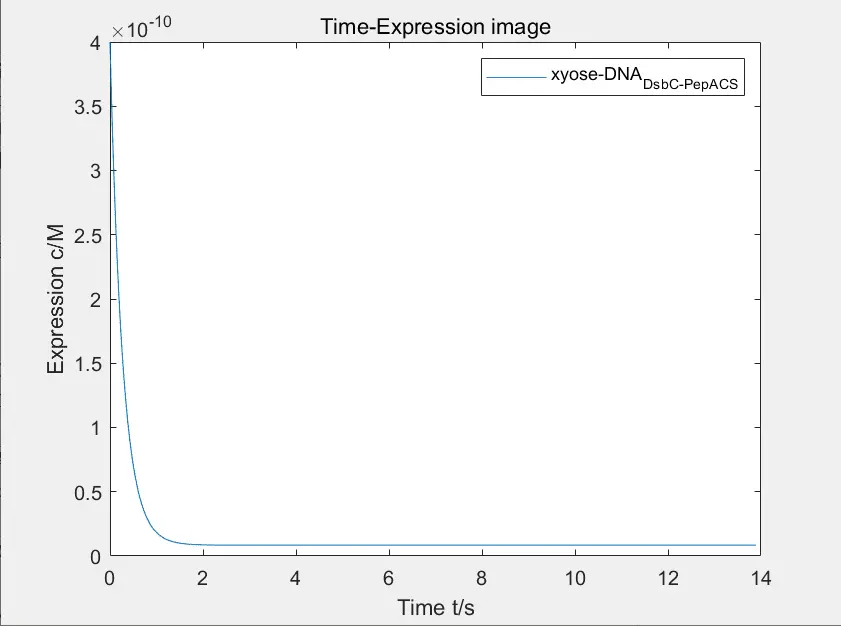Figure 10: the change of concentration of DNA bound to xylose

Due to the poor binding ability between regulatory factors and promoters, the desired function of using disulfide isomerase to straighten the hair cannot be realized. Our team strictly screened $$P_{YNR071C}$$ to carry out experiment.Amazing! No wonder the HUST-China got successful result in wet lab!

Hey, now I am thinking, it would be even better if there is an app to predict my hair color before dye. Have you ever dreamt of this kind of software?